## A 2.00-nF parallel-plate capacitor is charged to an initial potential difference AV}: 100 V and is then isolated. The dielectric mater

Question

A 2.00-nF parallel-plate capacitor is charged to an initial potential
difference AV}: 100 V and is then isolated. The dielectric material
between the plates is mica, with a dielectric constant of 5.00. (a) How
much work is required to withdraw the mica sheet? (b) What is the
potential difference across the capacitor after the mica is withdrawn?

in progress 0
5 months 2021-08-22T14:10:35+00:00 1 Answers 3 views 0

## Answers ( )

The answer to the question is;

(a) The amount of work required to withdraw the mica sheet is 4.0×10⁻⁵ J

(b) The potential difference across the capacitor after the mica is withdrawn is 500 V.

Explanation:

To solve the question, we note that

The Capacitance of the capacitor is proportional to the dielectric constant, that is removal of the dielectric material will reduce the capacitance of the capacitor by a factor of the dielectric constant as follows

Energy stored in a capacitor is given by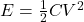Where:

C = Capacitance of the capacitor = 2.00 nF

V = Voltage = 100 V

Therefore before the dielectric material is removed, we have

E =×2.00 × 10⁻⁹×100² = 1.0×10⁻⁵ J

Asfter the dielectric material is removed we have

E = 5××2.00 × 10⁻⁹×100² = 5.0×10⁻⁵ J

Since energy is neither created nor destroyed, we have

Change in energy of system  = 5.0×10⁻⁵ J – 1.0×10⁻⁵ J = 4.0×10⁻⁵ J

Work done on the system to remove the mica sheet = energy change of the system = 4.0×10⁻⁵ J.

(b) From the equation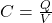Where

Q = Charge of the capacitor

V= Voltage

C = Capacitance of the capacitor, we have

Q, the charge of the capacitor is constant

and the final capacitance =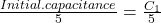Therefore we have

V₁ =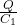V₂ =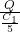=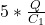= 5·V₁

The velocity increases by a factor of 5 and

V₂ = 5×V₁ =  5 × 100 V = 500 V.